# Ratio, Rates, & Unit Rates Lesson Plan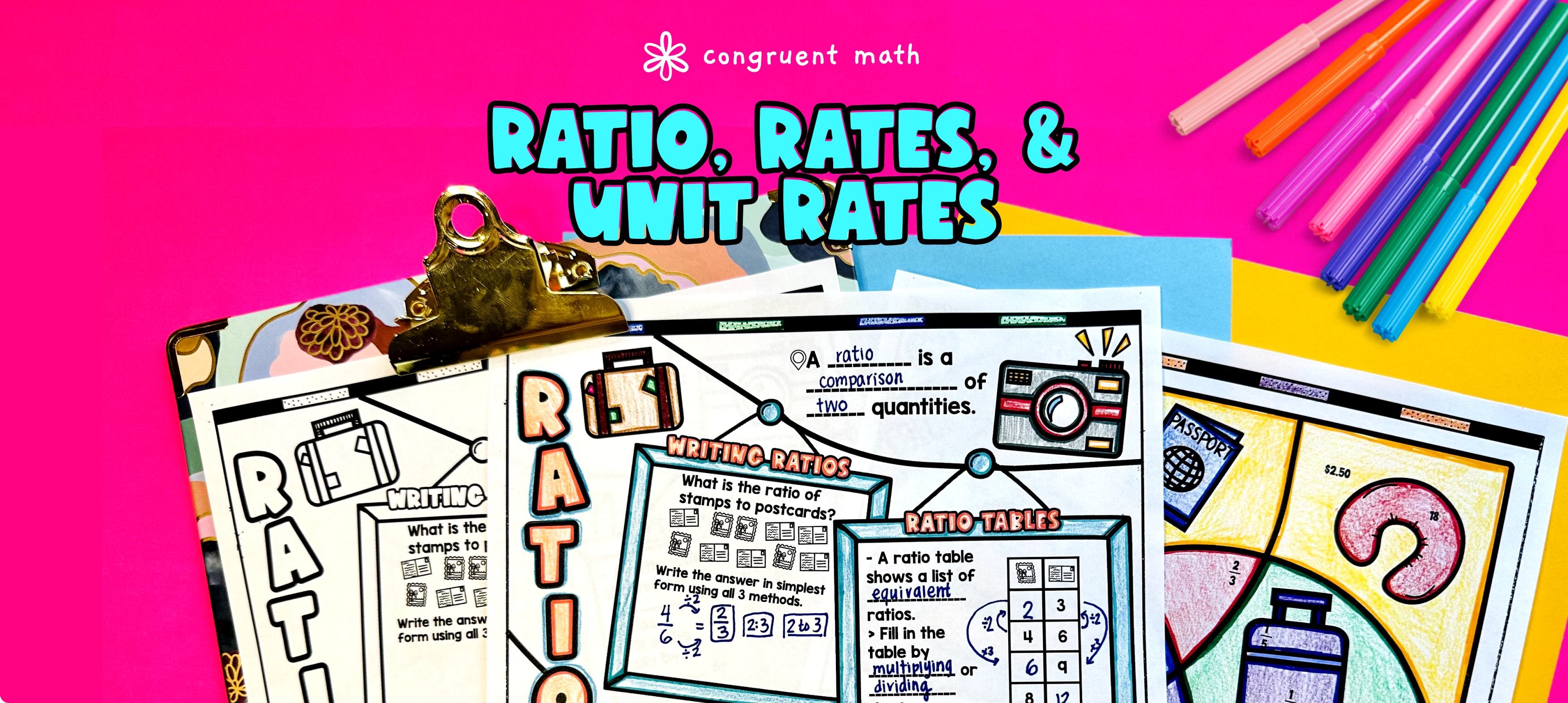Pin This

## Overview

Ever wondered how to teach ratios, rates, and unit rates in an engaging way to your 6th-grade students?

In this lesson plan, students will learn about ratios, rates, and unit rate and their real-life applications. Through artistic, interactive guided notes, with practice on writing ratios, filling out ratio tables to generate equivalent ratios and rates, calculating unit pricing, and word problems on ratios and rates. Also included is a color by number activity for students will gain a comprehensive understanding of the topic in a fun way. The lesson culminates with a real-life example that explores how unit pricing can be used to compare two products in the grocery store.

## Get the Lesson Materials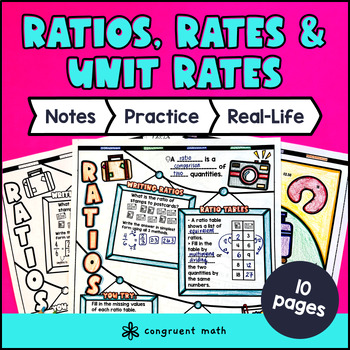\$4.25

## Learning Objectives

After this lesson, students will be able to:

• Define and explain ratios, rates, and unit rates

• Write ratios and rates in various forms

• Use ratio tables to generate equivalent ratios

• Calculate unit pricing

• Solve word problems involving ratios and rates

• Brainstorm real life applications of ratios and rates

## Prerequisites

Before this lesson, students should be familiar with:

• Basic multiplication and division skills

• Understanding of the concepts of factors and multiples

• Basic understanding of fractions and decimals (optional, but helpful)

## Key Vocabulary

• Ratio

• Rate

• Unit rate

• Equivalent ratios

• Ratio table

• Unit pricing

## Procedure

### Introduction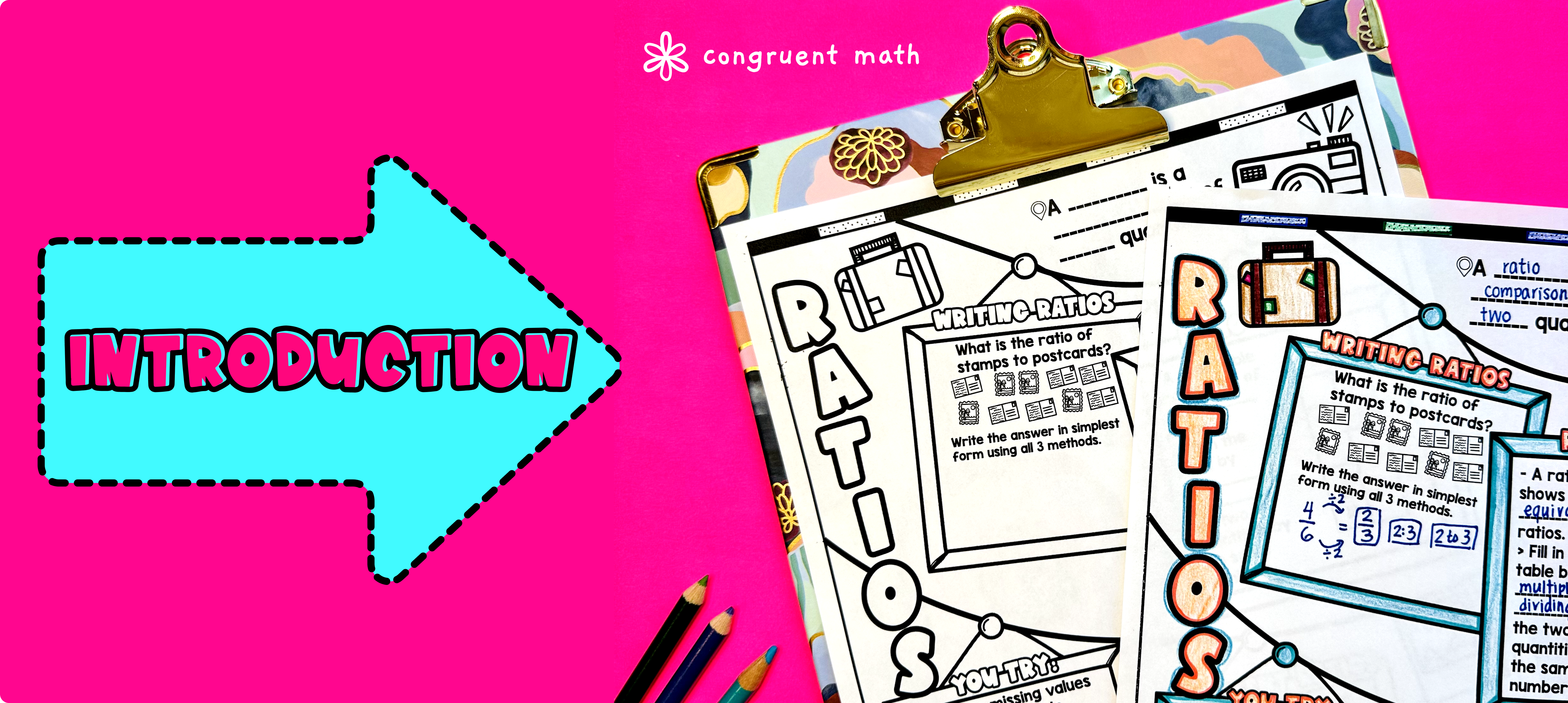Pin This

As a hook, ask students why unit pricing is important when shopping. Ask students if they ever had to comparison shop. What helped them to decide between several items? Refer to the last page of the guided notes as well as the FAQs below for ideas.

Use the guided notes to introduce the concepts of ratios, rates, and unit rates. Walk through the key points of each topic to teach. Refer to the FAQ below for a walk through on this, as well as ideas on how to respond to common student questions. Have students walk through the "Ratio, Rates, Unit Rates" notes section. Call on students to talk through their answers, potentially on the whiteboard or projector. Based on student responses, reteach concepts that students need extra help with.

### Check for Understanding & Practice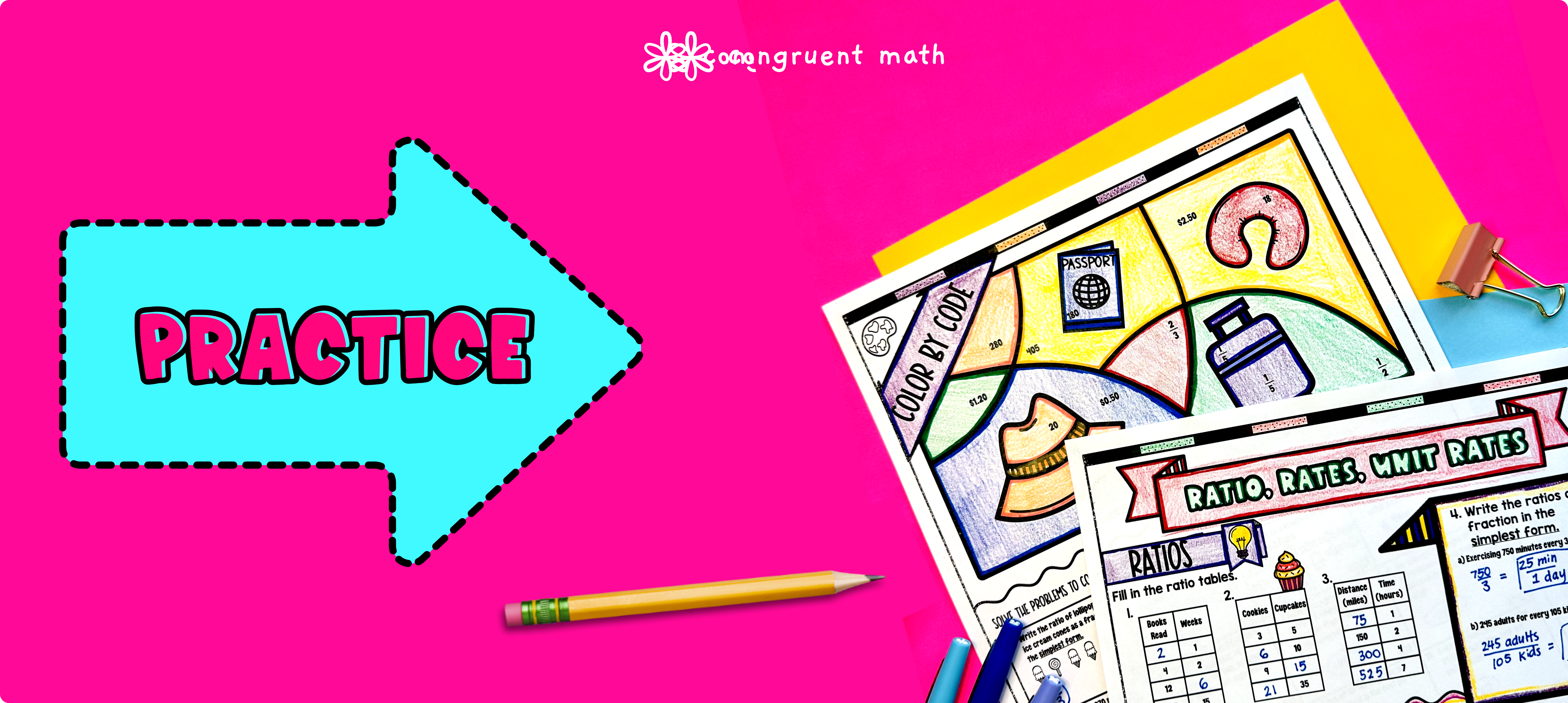Pin This

Have students work through the problems on the ratio, rates, unit rates practice sheet, either collaboratively or independently. Walk around and spot-check student answers on the check for understanding activity. Based on student responses, reteach concepts that students need extra help with. If your class has a wide range of proficiency levels, you can pull out students for reteaching, and have more advanced students begin work on the practice exercises.

Then, have students practice the concepts of ratios, rates, and unit rates using the Color by Number activity. The activity includes a variety of problems, including writing ratios, calculating unit pricing, and solving word problems on ratios and rates. Walk around the room to answer student questions and check for understanding.

Fast finishers can dive into the Real-Life Application section of this lesson plan to explore how unit pricing can be used to compare two products in the grocery store. You can assign it as homework for the remainder of the class.

### Real-Life Application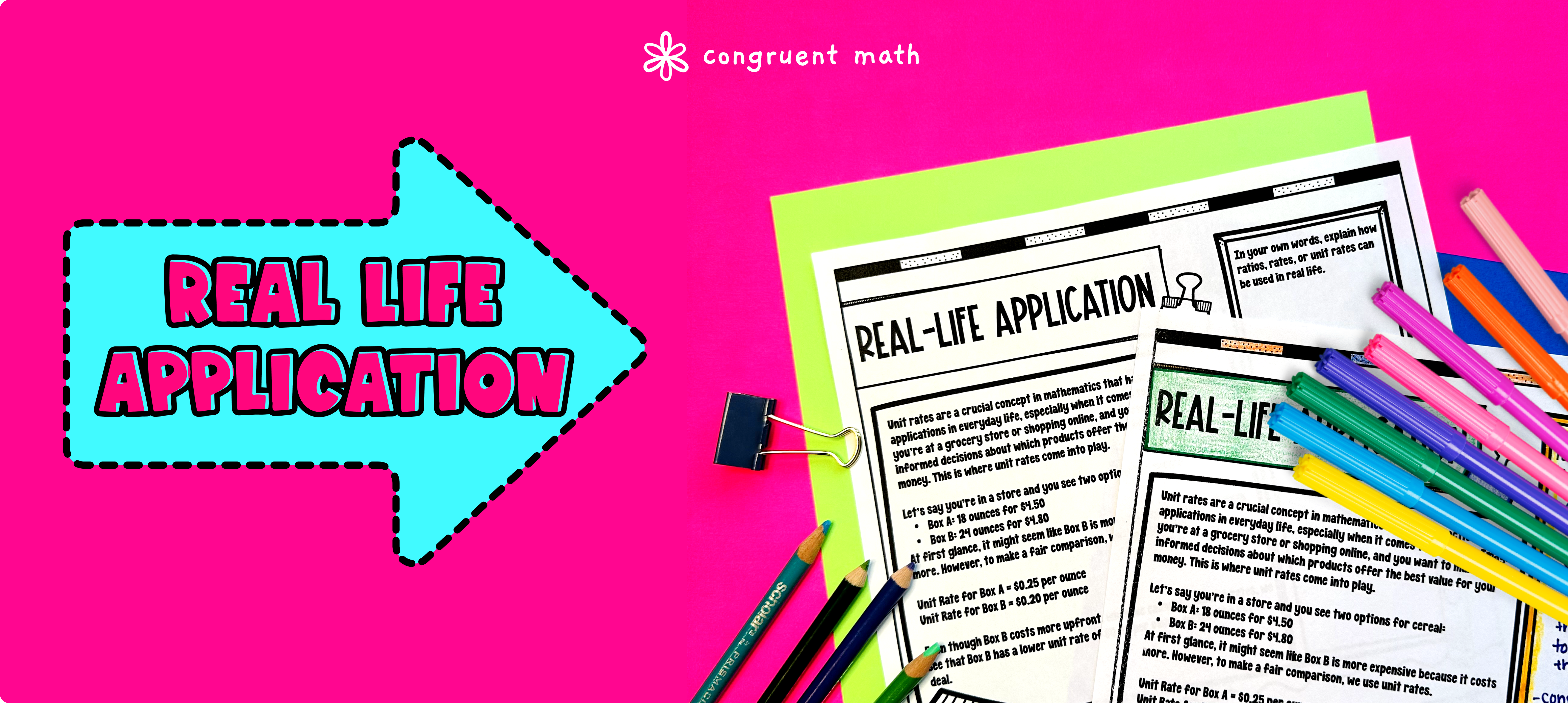Pin This

Bring the class back together, and introduce the concept of unit pricing as a tool for comparing the cost of two products in the grocery store. Explain and hold a discussion on how unit pricing can help consumers make informed decisions about which products to purchase.

Have students work in small groups to compare the unit pricing of two similar products, such as two brands of cereal or two sizes of juice. After completing the activity, have students share their findings with the class and discuss any trends or patterns they noticed. Refer to the FAQs for more ideas on how to teach this concept!

## Extensions

**If you're looking for digital practice for ratios, rates, and unit rates, try my Pixel Art activities in Google Sheets. Every answer is automatically checked, and correct answers unlock parts of a mystery picture. It's incredibly fun, and a powerful tool for differentiation.

Here are 4 self checking Google Sheets explore: